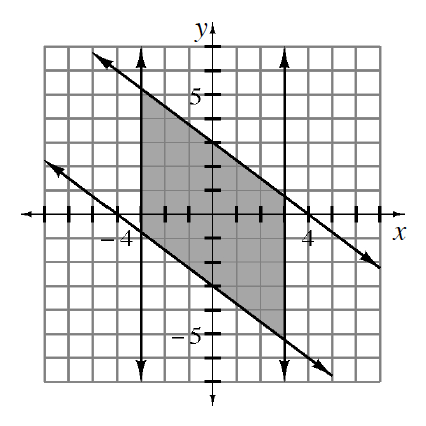### Home > CCA2 > Chapter Ch6 > Lesson 6.2.4 > Problem6-147

6-147.

Write a system of inequalities that could be represented by the graph at right.

Start by finding an equation for each line, and then test points to determine which inequality to use.

One of the inequalities is: $y\le-\frac{3}{4}x+3.$